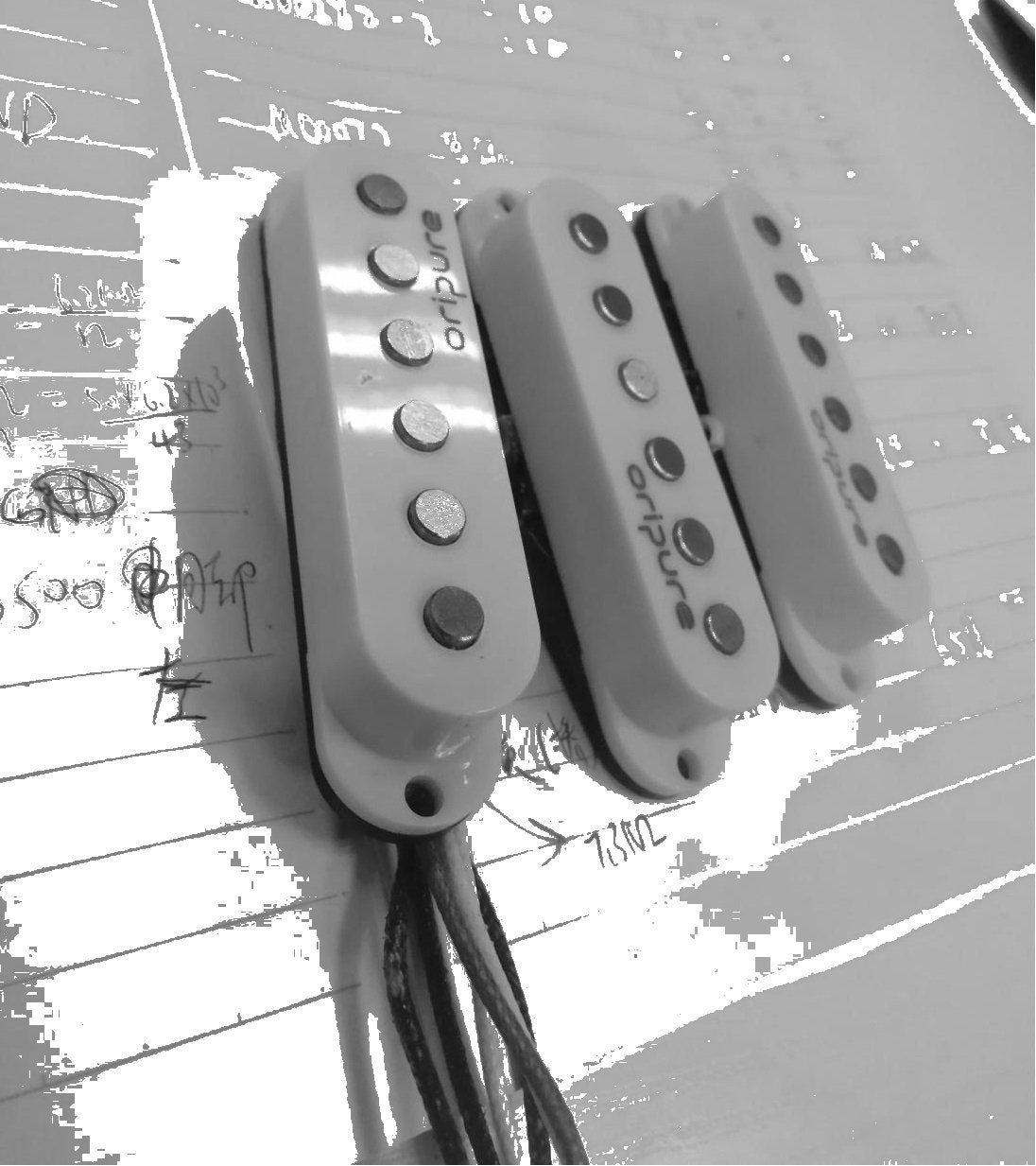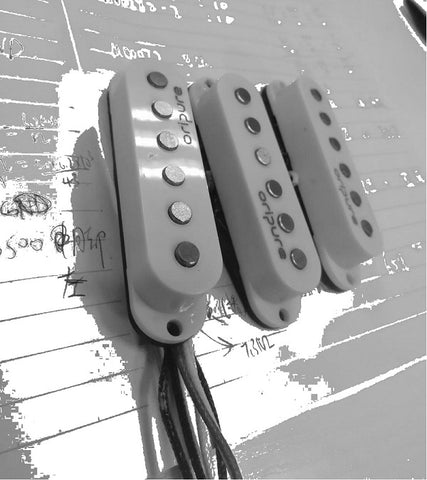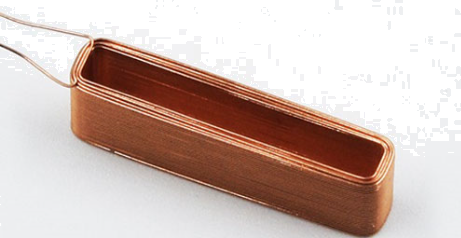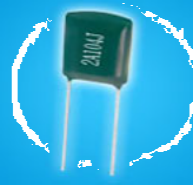# Explore the little secrets of electric guitar pickups (1)

In this article, I will talk about how to judge the general trend of the pickup tone through some intuitive parameter values.We can measure three parameters for a single pickup: resistance, capacitance, and inductance.

First, the structure of the pickup is an RLC resonant circuit, and its output is closely related to the value of RLC.

The formula for resonance frequency is F=1/(2Π√LC)

Here is a brief explanation about the resonant frequency: the resonant frequency refers to a circuit containing a capacitor and an inductor. If the capacitor and the inductor are connected in parallel, it may appear in a short period of time: the voltage of the capacitor gradually increases, but the current Decrease gradually; the inductor current gradually increases, but the inductor voltage gradually decreases. And in another short period of time: the voltage of the capacitor gradually decreases, but the current gradually increases; the current of the inductor gradually decreases, but the voltage of the inductor gradually increases. The increase in voltage can reach a positive maximum value, and the decrease in voltage can also reach a negative maximum value. The direction of the current will also change in positive and negative directions during this process, which is called the electrical oscillation of the circuit. When the sinusoidal frequency of the external input voltage of the circuit reaches a certain frequency (that is the resonant frequency of the circuit), the inductance and capacitive reactance of the resonant circuit are equal, Z=R, and the resonant circuit is purely resistive to the outside, which is resonance.

1. Inductance

In fact, whether it is the number of turns of the coil, the thickness of the coil, the strength of the magnet, etc., the effect on the output is concluded as the effect of the inductance. For example, it becomes one coil to work when we perform a single cut, the inductance is reduced. At this time, it will also sound soft and transparent. Then the output sound is also a little smaller when performing on double coils.

The increase in the number of turns and the thickness of the coil will increase the inductance. For example, the output of ceramic magnets is larger than that of magnetic steel magnets, since the ceramic magnets have greater inductance.

From the formula, we can see that the larger the inductance L, the smaller the resonance frequency F. As the inductance increases, the effect is reduced high-frequency signals, thicker low-frequency signals, and strong output. On the contrary, if the inductance is reduced, the tone will be more transparent and brighter.1. Resistance

The resistance here refers to the DC resistance of the coil. This parameter can roughly reflect the inductance and the state of the coil. As the resistance increases, the output will become stronger, which is basically the same as the effect of inductance.

1. Capacitance

Capacitors will affect the attenuation of high frequency. We know that capacitors will filter out high-frequency signals, increase the capacitance, and reduce the resonance frequency.To sum it up, there is no standard for these values. Different parameters will have different effects. There is a specific analysis for the specific situation, but a general trend can still be judged from it.